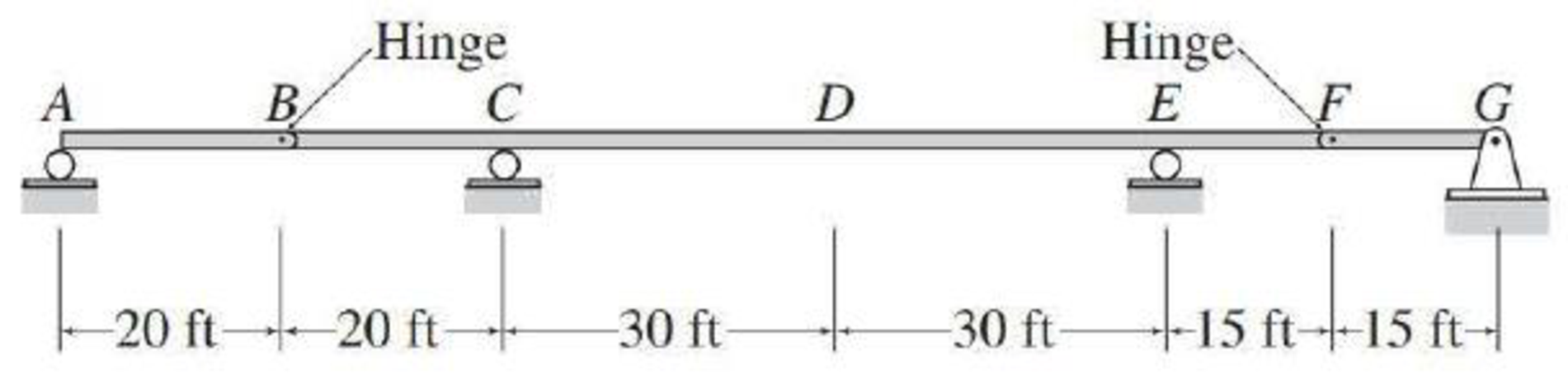# For the beam of Problem 8.23, determine the maximum positive and negative shears and the maximum positive and negative bending moments at point D due to a concentrated live load of 30 k, a uniformly distributed live load of 3 k/ft, and a uniformly distributed dead load of 1 k/ft. FIG. P8.23, P8.25

#### Solutions

Chapter
Section
Chapter 9, Problem 7P
Textbook Problem
6 views

## For the beam of Problem 8.23, determine the maximum positive and negative shears and the maximum positive and negative bending moments at point D due to a concentrated live load of 30 k, a uniformly distributed live load of 3 k/ft, and a uniformly distributed dead load of 1 k/ft.FIG. P8.23, P8.25

To determine

Draw the influence lines for the shear and bending moment at point D.

### Explanation of Solution

Given Information:

The concentrated live load (P) is 30 k.

The uniformly distributed live load (wL) is 3 k/ft.

Calculation:

Use Muller-Breslau's principle.

Influence line for the shear at point D.

Cut the beam at D to obtain the released structure of the beam. Next apply a small relative displacement in positive direction of SD by moving end D of the portion CD downward by Δ1 and end D of the portion ED upward by Δ2 to obtain the deflected shape.

Sketch the deflected shape of the released beam when the beam cut at D as shown in Figure 1.

The general shape of the influence line for shear (SD) at D is the similar of deflected shape.

Sketch the general shape of the influence line for shear (SD) at D as shown in Figure 2.

Find the numerical value of influence line ordinate at D.

Place the 1 k load first just to the left of D and then just to the right of D.

Sketch the free body diagram of beam as shown in Figure 3.

Find the vertical reaction (Ay) at A.

Consider moment at B from A.

Consider clockwise moment as positive and anticlockwise moment as negative.

ΣMB=0Ay×20=0Ay=0

Find the vertical reaction (Gy) at G.

Consider moment at F from G.

Consider clockwise moment as negative and anticlockwise moment as positive.

ΣMF=0Gy×20=0Gy=0

Find the vertical reactions Cy and Ey.

Consider moment at B from G.

Consider clockwise moment as negative and anticlockwise moment as positive.

ΣMB=0Gy(110)+Ey(80)1(50)+Cy(20)=0

Substitute 0 for Gy.

(Gy)(110)+Ey(80)1(50)+Cy(20)=080Ey+20Cy=50        (1)

Consider moment at F from A.

Consider clockwise moment as positive and anticlockwise moment as negative.

ΣMF=0Ay(115)+Cy(75)1(45)+Ey(15)=0

Substitute 0 for Ay.

(0)(115)+Cy(75)1(45)+Ey(15)=075Cy+15Ey=45        (2)

Solve Equations (1) and (2),

Cy=0.5kEy=0.5k

Find the shear at D (SD,L) when unit load 1 k placed just left of D using the equation:

SD,L=Ey

Substitute 0.5 k for Ey.

SD,L=0.5k

Thus, the value of influence line ordinate just left of D is ‑0.5 k/k.

Find the shear at D (SD,R) when unit load 1 k placed just right of D using the equation:

SD,R=Cy

Substitute 0.5 k for Cy.

SD,R=0.5k

Thus, the value of influence line ordinate just right of D is 0.5 k/k.

The numerical values of influence line ordinate in different points on the beam for shear SD at D are determined using geometry (similar triangle) of the influence line and summarize the values as in Table 4.

 x (ft) Points Influence line ordinate of SD (k/k) 0 A 0 20 B 13 40 C 0 70 D− −12 70 D+ 12 100 E 0 115 F −14 130 G 0

Sketch the influence line diagram for shear at point D as shown in Figure 4.

Refer Figure 4.

The maximum positive ILD ordinate is 12k/k.

The maximum negative ILD ordinate is 12k/k.

Find the positive area (A1) of the influence line diagram of shear force at point D.

A1=12(LAC)(13)+12(LDE)(12)

Here, LAC is the length of beam between A to C and LDE is the length of beam between D to E.

Substitute 40 ft for LAC and 30 ft for LDE.

A1=12(40)(13)+12(30)(12)=6.667+7.5=14.167ft

Find the negative area (A2) of the influence line diagram of shear force at point D.

A2=12(LCD)(12)+12(LEG)(14)

Here, LCD is the length of beam between C to D and LEG is the length of beam between E to G.

Substitute 30 ft for LCD and 30 ft for LEG.

A2=12(30)(12)+12(30)(14)=7.53.75=11.25ft

Find the maximum positive shear at point D using the equation.

Maximum positive SC=P(maximum positive ILD ordinate)+wL(A1)+wD(A1+A2)

Substitute 30 k for P, 12k/k for maximum positive ILD ordinate, 3 k/ft for wL, 14.167ft for A1, 1 k/ft for wD, and 11

### Still sussing out bartleby?

Check out a sample textbook solution.

See a sample solution

#### The Solution to Your Study Problems

Bartleby provides explanations to thousands of textbook problems written by our experts, many with advanced degrees!

Get Started

Find more solutions based on key concepts
The thermal conductivity of a solid material can be determined using a setup similar to the one shown in the ac...

Engineering Fundamentals: An Introduction to Engineering (MindTap Course List)

What are the three primary types of public law?

Management Of Information Security

List six steps involved in performing a visual inspection.

Automotive Technology: A Systems Approach (MindTap Course List)

What does the word, live, signify when used to describe a type of media (i.e., Live USB or Live CD)? (413)

Enhanced Discovering Computers 2017 (Shelly Cashman Series) (MindTap Course List)

What is (are) the major alloying element(s) of 6061 aluminum?

Precision Machining Technology (MindTap Course List)

If your motherboard supports ECC DDR3 memory, can you substitute non-ECC DDR3 memory?

A+ Guide to Hardware (Standalone Book) (MindTap Course List)

Which electrodes would be grouped in the following F numbers: B, F2, F4?

Welding: Principles and Applications (MindTap Course List)